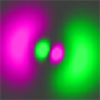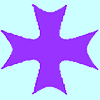# Resources tagged with: Polar coordinates

Filter by: Content type:
Age range:
Challenge level:

### There are 5 results

Broad Topics > Coordinates, Functions and Graphs > Polar coordinates### Polar Bearings

##### Age 16 to 18 Challenge Level:

What on earth are polar coordinates, and why would you want to use them?### An Introduction to Polar Coordinates

##### Age 14 to 18

This introduction to polar coordinates describes what is an effective way to specify position. This article explains how to convert between polar and cartesian coordinates and also encourages the. . . .### Maltese Cross

##### Age 16 to 18 Challenge Level:

Sketch the graph of $xy(x^2 - y^2) = x^2 + y^2$ consisting of four curves and a single point at the origin. Convert to polar form. Describe the symmetries of the graph.### Polar Flower

##### Age 16 to 18 Challenge Level:

This polar equation is a quadratic. Plot the graph given by each factor to draw the flower.### Bent Out of Shape

##### Age 14 to 18 Challenge Level:

An introduction to bond angle geometry.